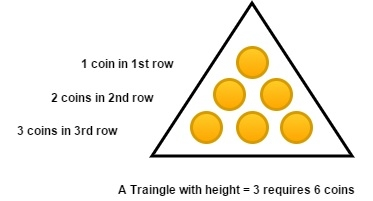# C/C++ Program for Number of solutions to Modular Equations?

CC++Server Side ProgrammingProgramming

We have an n number of coins and we have to French the coin way that it makeup Pyramid of maximum height. We will arrange the first coin in First row second and third coin in the second row and so onIn the given diagram, we make pyramid 6 of coins having a height of 3. We cannot make height 4 but we will need 10 coins. It is simple to get the height by using this formula;

H = {(-1+ √(1+8N))/2}

Input: n = 10
Output: Height of pyramid: 4

## Explanation

Height by using this formula

H = {(-1+ √(1+8N))/2}

## Example

#include <iostream>
#include <math.h>
using namespace std;
int main() {
int n=10;
int height = (-1 + sqrt(1 + 8 * n)) / 2;
cout << "Height of pyramid: " <<height;
}